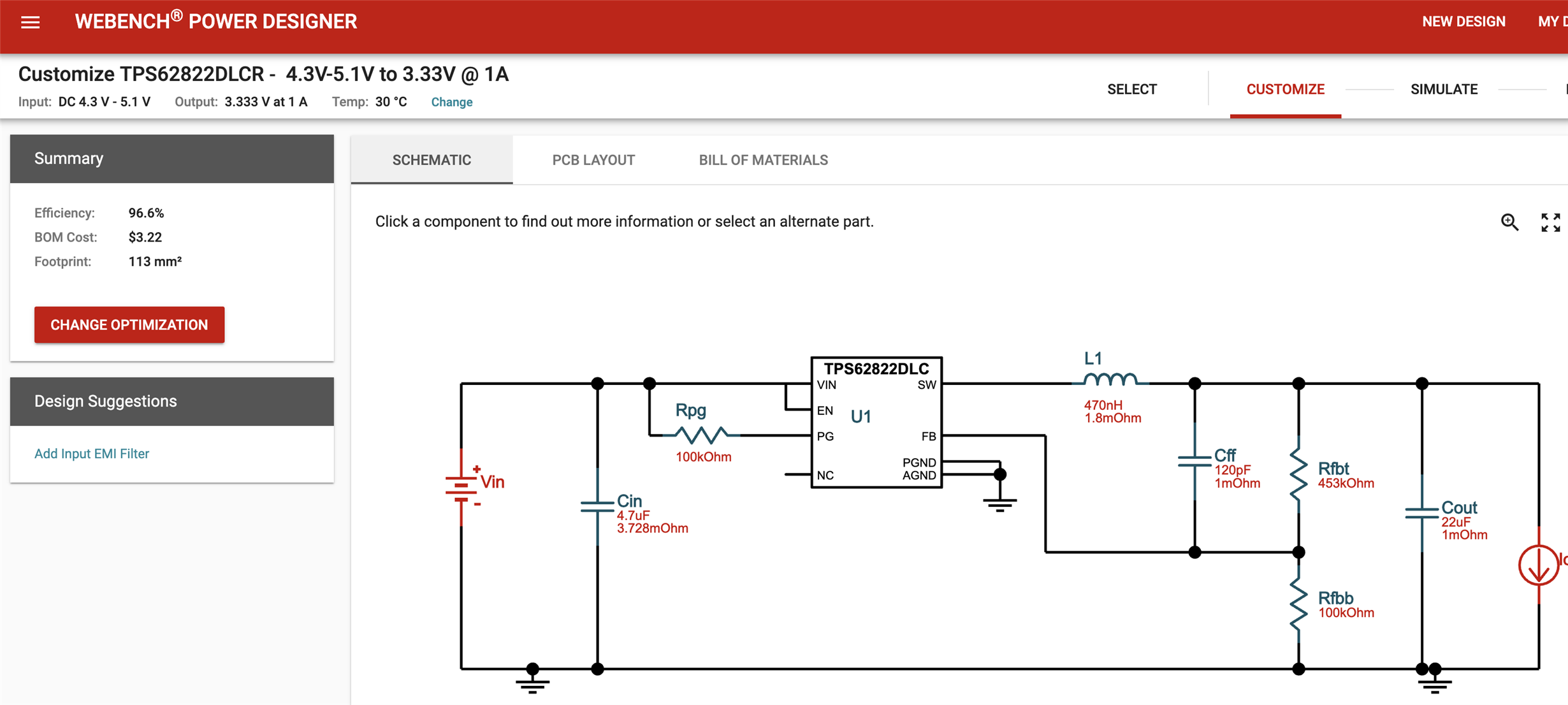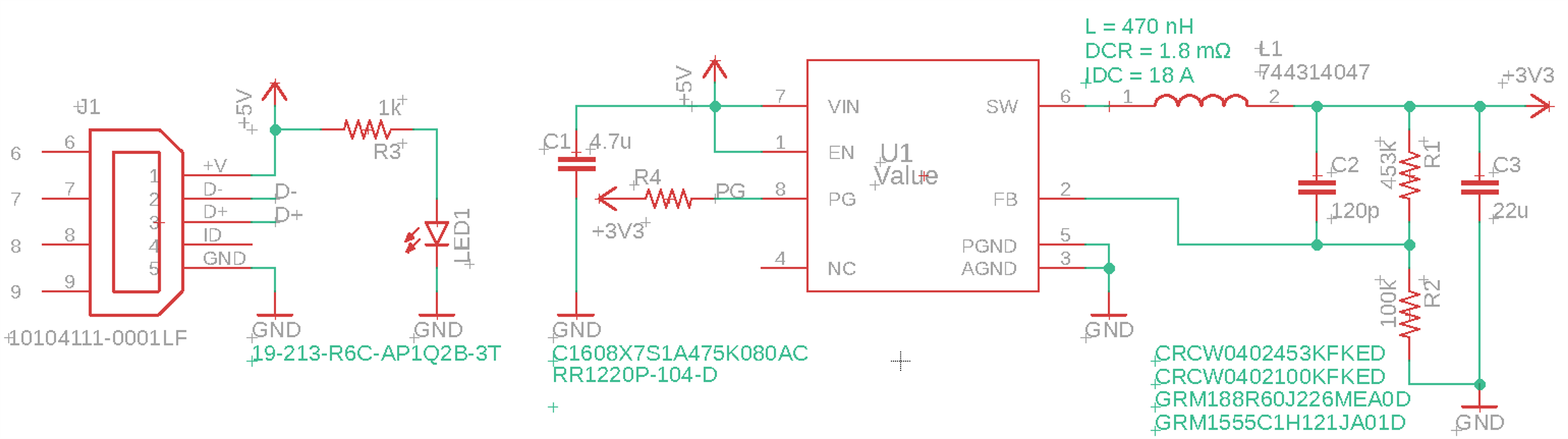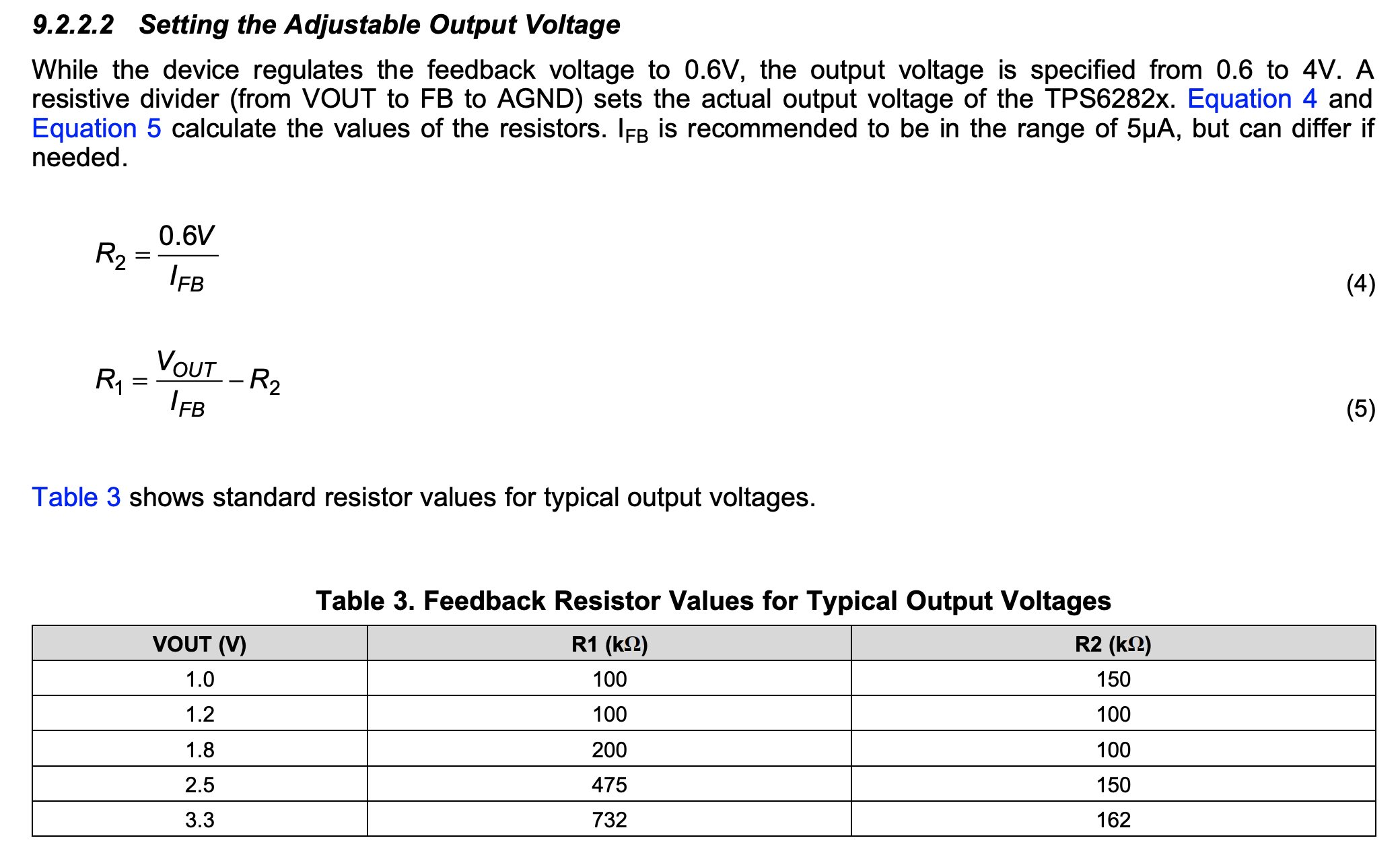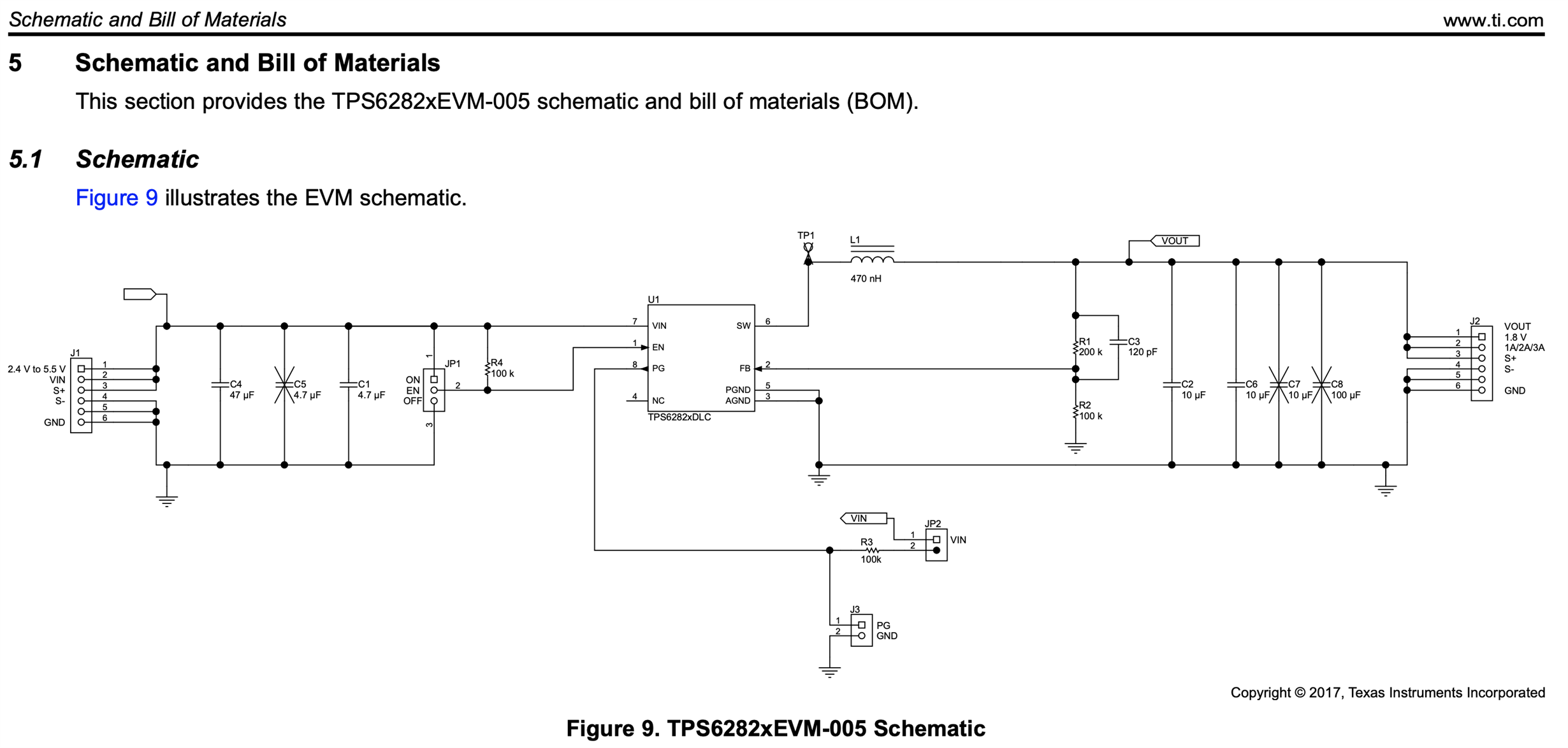If you have a related question, please click the "Ask a related question" button in the top right corner. The newly created question will be automatically linked to this question.

# TPS62821: I_FB, PG pull-up setting

Part Number: TPS62821

Hi, guys. I'm thinking of using the TPS62821 to provide 3.3V to power up the MSP432 MCU. I'm creating a custom PCB that uses 5V micro USB power. Thanks to the WEBENCH tool,I referred this and created my schematic using EAGLE 9.4.The main difference is that I connected the VOUT 3.3V to the pull-up resistor to hold the PG(Power Good) pin, not using the VIN because I wanted to check the voltage level using the MSP432.

The WEBENCH tool was lovely, but I got confused with the datasheet, which mentions about the I_FB.I want to get the 3.3V (Vout Actual = 3.32V). From Table 3, R1 = 732k, R2 = 162k. Whereas, the WEBENCH recommended R1 = 453k, R2 = 100k.

Q0. I cannot see many details related to I_FB, only seeing that this should have a range of 5uA.

Although this looks like the current of the feedback resistor, may I ask what is I_FB?

Q1. According to equation 4 and using R2 = 100k, I_FB = 6uA. Is this in the "range of 5uA"? I cannot find the margins for I_FB in the datasheet.

Q2. I'm curious why I got a different suggestion compared to Table 3. (R1 = 732k, R2 = 162k VS R1 = 453k, R2 = 100k)

Is there a difference (getting lower current when choosing R1 = 453k, R2 = 100k for instance) between these pairs? I just want to know whether this combination is correct/better.

Q3. I want to use PG to toggle between 3.3V and 0V so that I can use this as an interrupt source for the MSP432. In this case, connecting the pull-up resistor with 3.3V VOUT is correct, right?

• Hello,
in the D/S is specified only the leakage current at the input of the FB (max is around 50nA). What is reported in the D/S as I_FB is the current flowing through the feedback divider that needs to be provided not to affect the output (to be consistent, is three orders of magnitude higher than the leakage current at the FB pin). In table 3, there is an example of the resistors that the customer could use to reach the desired output. What is shown in the webench is anyway correct, it will flow more current (above 5uA), and it is ok.
In the following link are shown different considerations regarding the resistive feedback divider.
www.ti.com/.../slyt469.pdf
In some cases, reduce the value of the resistors in the feedback divider may improve the noise sensitivity. On the other hand, use a different value for R1 and R2, will change the position of the pole and zero associated with the Cff. Both considerations are explained in the attached link.
• Thanks, Alfonso.

I forgot to ask; about the PG tied to 3.3V, is it okay?

• Hello,
sorry I didn't write before the reply to your last question. Yes, you can connect it to 3.3V.